#### The ideal distance function

The ideal way to define distance onis to use a cost functional and then define the distance from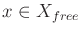to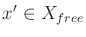as the optimal cost-to-go fromto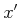while remaining in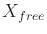. In some cases, it has been also referred to as the nonholonomic metric, Carnot-Caratheodory metric, or sub-Riemannian metric . Note that this not a true metric, as mentioned in Section 5.1.2, because the cost may not be symmetric. For example, traveling a small distance forward with Dubins car is much shorter than traveling a small distance backward. If there are obstacles, it may not even be possible to reach configurations behind the car.

This concept of distance should be somewhat disturbing because it requires optimally solving the motion planning problem of Formulation 14.1. Thus, it cannot be practical for efficient use in a motion planning algorithm. Nevertheless, understanding this ideal notion of distance can be very helpful in designing practical distance functions on. For example, rather than using a weighted Euclidean metric (often called Mahalanobis metric) for the Dubins car, a distance function can be defined based on the length of the shortest path between two configurations. These lengths are straightforward to compute, and are based on the optimal curve families that will be covered in Section 15.3. This distance function neglects obstacles, but it should still provide better distance information than the weighted Euclidean metric. It may also be useful for car models that involve dynamics.

The general idea is to get as close as possible to the optimal cost-to-go without having to perform expensive computations. It is often possible to compute a useful underestimate of the optimal cost-to-go by neglecting some of the constraints, such as obstacles or dynamics. This may help in applying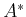search heuristics.

Steven M LaValle 2020-08-14